# Orlík hydroelectric plant

The Orlík hydroelectric power plant, built in 1954-1961, consists of four Kaplan turbines. For each of them, the water with a flow rate of Q = 150 m3/s is supplied with a flow rate of h = 70.5 m at full power.
a) What is the total installed power of the power plant at efficiency n = 87%?
b) For the fast charging of electric vehicles overnight, power consumption up to P0 = 11kW is required. How many would electric cars cover power plant power?
c) How many days of full power operation corresponds to the delivered energy E = 398GWh

Result

P =  361.018 MW
n =  32820
d =  45.9

#### Solution:Leave us a comment of example and its solution (i.e. if it is still somewhat unclear...):

Showing 0 comments:Be the first to comment!#### To solve this example are needed these knowledge from mathematics:

Do you know the volume and unit volume, and want to convert volume units? Do you want to convert mass units?

## Next similar examples:

1. Elevator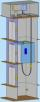In homes with more floor elevators are used. For passenger transport, the most commonly used traction elevator counterweight. The top of the shaft engine room with the engine. The car is suspended on a rope, which is guided up over two pulleys to the count
2. Cylindrical tank 2If a cylindrical tank with volume is used 12320cm raised to the power of 3 and base 28cm is used to store water. How many liters of water can it hold?
3. Solid in waterThe solid weighs in air 11.8 g and in water 10 g. Calculate the density of the solid.
4. CarAt what horizontal distance reaches the car weight m = 753 kg speed v = 74 km/h when the car engine develops a tensile force F = 3061 N. (Neglect resistance of the environment.)
5. Shot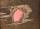Shot with a mass 43 g flying at 256 m/s penetrates into the wood to a depth 25 cm. What is the average force of resistance of wood?
6. Water flowHow much water flow in pipe with a diameter of 16 cm in 1 hour if the water velocity is 2.5 m/s?
7. G forcesCalculate deceleration of car (as multiple of gravitational acceleration g = 9.81 m/s2) which occurs when a car in a frontal collision slows down uniformly from a speed 111 km/h to 0 km/h in 1.2 meters trajectory.
8. Aircraft nose downHow long will fall airliner from a height of 10000 m at speed 1,000 km/h?
9. Iron cast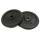What is the weight of cast iron with volume 3575 cubic centimeters. The density of the cast iron is 7600 kg/m3
10. FlywheelFlywheel turns 450 rev/min (RPM). Determine the magnitude of the normal acceleration of the flywheel point which are at a distance of 10 cm from the rotation axis.
11. Cuprum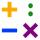From 1600 mm long sheet of copper plate 2 mm thickness we have separated over the whole length the belt weighing 6000 g. Calculate belt width if 1 dm3 copper weighs 8.9 kg.
12. GasolineHow many liters of gas is in the tank if its weight 15.4kg. Specific density of gasoline is 770kg/m3.
13. BomberThe aircraft flies at an altitude of 4100 m above the ground at speed 777 km/h. At what horizontal distance from the point B should be release any body from the aircraft body to fall into point B? (g = 9.81 m/s2)
14. Bulb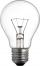A 60 W bulb consumes 0.12 kWh of electricity. How long did she stand light without a break?
15. Clock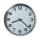How many hours are 15 days?
16. Water level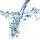To cuoid shaped poll, bottom size 2m and 3.5m; flows water at a rate of 50 liters per minute. How long will take to water reach level 50 cm?
17. The shopThe shop has 3 hectoliters of water. How many liter bottles is it?